Students can Download Computer Science Chapter 14 Classes and Objects Questions and Answers, Notes Pdf, Samacheer Kalvi 11th Computer Science Book Solutions Guide Pdf helps you to revise the complete Tamilnadu State Board New Syllabus and score more marks in your examinations.

## Tamilnadu Samacheer Kalvi 11th Computer Science Solutions Chapter 14 Classes and Objects

### Samacheer Kalvi 11th Computer Science Classes and Objects Text Book Back Questions and Answers

PART – 1

Question 1.
The variables declared inside the class are known as data members and the functions are known as ………………..
(a) data functions
(b) inline functions
(c) member functions
(d) attributes
(c) member functions

Question 2.
Which of the following statements about member functions are True or False?
(i) A member function can call another member function directly with using the dot operator.
(ii) Member function can access the private data of the class.
(a) (i) – True, (ii) – True
(b) (i) – False, (ii)-True
(c) (i) – True, (ii) – False
(d) (i) – False, (ii) – False
(a) (i) – True, (ii) – True

Question 3.
A member function can call another member function directly, without using the dot operator called as ………………..
(a) sub function
(b) sub member
(c) nesting of member function
(d) sibling of member function
(c) nesting of member functionQuestion 4.
The member function defined within the class behave like ………………..
(a) inline function
(b) Non – inline function
(c) Outline function
(d) Data function
(a) inline function

Question 5.
Which of the following access specifier protects data from inadvertent modifications?
(a) Private
(b) Protected
(c) Public
(d) Global
(b) Protected

Question 6.
class x
{
int y; public:
x(int z){y=z;}
}x1;
int main()
{x x2(10);
return 0;}
How many objects are created for the above program?
(a) 10
(b) 14
(c) 5
(d) 2
(c) 5

Question 7.
State whether the following statements about the constructor are True or False.
(i) constructors should be declared in the private section.
(ii) constructors are invoked automatically when the objects are created.
(a) True, True
(b) True, False
(c) False, True
(d) False, False
(c) False, TrueQuestion 8.
Which of the following constructor is executed for the following prototype?
(a) Default constructor
(b) Parameterized constructor
(c) Copy constructor
(d) Non Parameterized constructor
(d) Non Parameterized constructor

Question 9.
What happens when a class with parameterized constructors and having no default constructor is used in a program and we create an object that needs a zero – argument constructor?
(a) Compile – time error
(b) Domain error
(c) Runtime error
(d) Runtime exception
(d) Runtime exception

Question 10.
Which of the following create a temporary instance?
(a) Implicit call to the constructor
(b) Explicit call to the constructor
(c) Implicit call to the destructor
(d) Explicit call to the destructor
(b) Explicit call to the constructor

PART – 2
II. Answers to all the questions

Question 1.
What are called members?
Class comprises of members. Members are classified as Data Members and Member functions. Data members are the data variables that represent the features or properties of a class. Member functions are the functions that perform specific tasks in a class.

Question 2.
Differentiate structure and class though both are user defined data type.
The only difference between structure and class is the members of structure are by default public where as it is private in class.Question 3.
What is the difference between the class and object in terms of OOP?
Object:

• Object is an instance of a class.
• Object is a real world entity such as pen, laptop, mobile, chair etc.
• Object allocates memory when it is created.

Class:

• Class is a blueprint or template from which objects are created.
• Class is a group of similar objects.
• Class doesn’t allocate memory when it is created.

Question 4.
Why it is considered as a good practice to define a constructor though compiler can automatically generate a constructor?
Declaring a constructor with arguments hides the compiler generated constructor. After this we cannot invoke the compiler generated constructor.

Question 5.
Write down the importance of destructor.
The purpose of the destructor is to free the resources that the object may have acquired during its lifetime. A destructor function removes the memory of an object which was allocated by the constructor at the time of creating a object.

PART – 3
III. Answers to all the questions

Question 1.
Rewrite the following program after removing the syntax errors if any and underline the errors: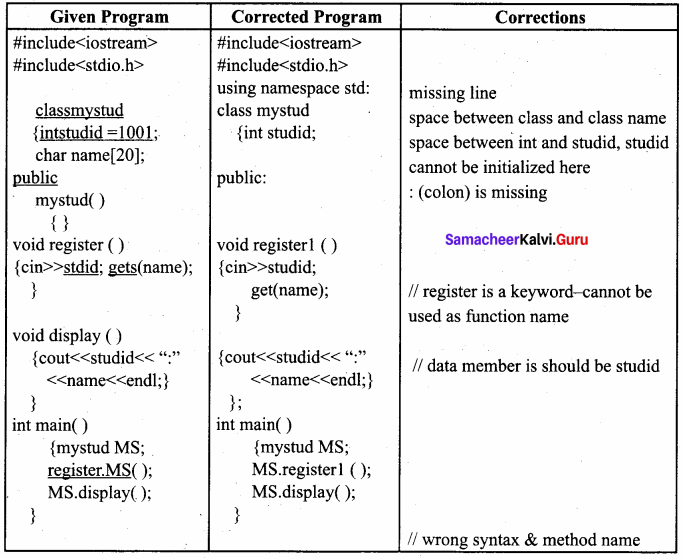Question 2.
Write with example how will you dynamically initialize objects?
When the initial values are provided during runtime then it is called dynamic initialization.
Example to illustrate dynamic initialization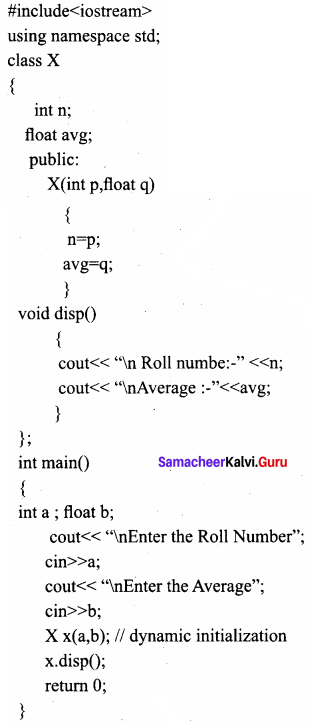Output:
Enter the Roll Number 1201
Enter the Average 98.6
Roll number:- 1201
Average :- 98.6

Question 3.
What are advantages of declaring constructors and destructor under public accessability?
When constructor and destructor are declared under public:

1. we can initialize the object while declaring it.
2. we can explicitly call the constructor.
3. we can overload constructor and therefore use multiple constructors to initialize objects automatically.
4. we can destroy the objects at the end of class scope automatically (free unused memory).

However, some C++ compiler and Dev C++ do not allow to declare constructor and destructor under private section. So it is better to declare constructor and destructor under public section only.

Question 4.
Given the following C++ code, answer the questions (i) & (ii).
class TestMeOut
{

public:
~TestMeOut() //Function 1 {cout << “Leaving the examination hall” << endl;}
TestMeOut() //Function 2
{cout<<“Appearing for examination”<<endl;}
void MyWork() //Function 3
{cout<<“Attempting Questions//<<endl;}

};
1. In Object Oriented Programming, what is Function 1 referred as and when does it get invoked / called?
2. In Object Oriented Programming, what is Function 2 referred as and when does it get invoked / called?
1. Function 1 is the ‘destructor’.
It is executed automatically when an object of the class TestMeOut goes out of scope.

2. Function 2 is called default ‘constructor’.
It is executed automatically when instance of the class TestMeOut comes into scope or when objects of the class TestMeOut are created.

Question 5.
Write the output of the following C++ program code :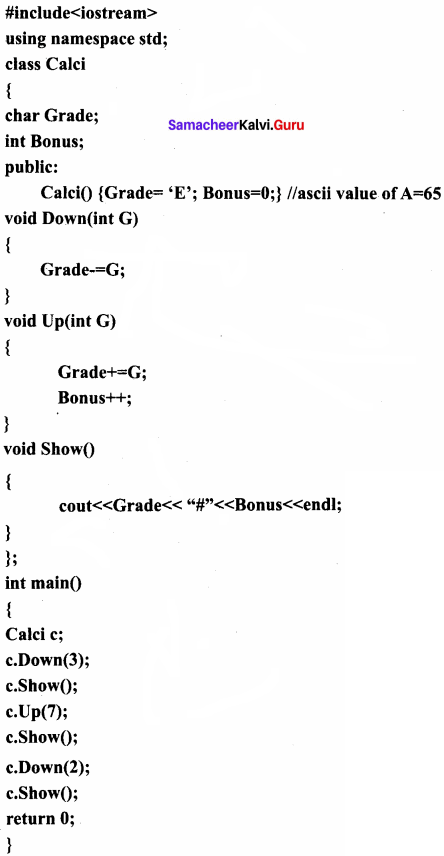Output:
B#0
I#1
G#1

PART – 4
IV. Answers to all the questions

Question 1.
Explain nested class with example.
When one class become the member of another class then it is called Nested class and the relationship is called containership. When a class is declared within another class, the inner class is called as Nested class (i.e. the inner class) and the outer class is known as Enclosing class. Nested class can be defined in private as well as in the public section of the Enclosing class.

Classes can be nested in two ways:

1. By defining a class within another class
2. By declaring an object of a class as a member to another class
3. By defining a class within another class

C++ program to illustrate the nested class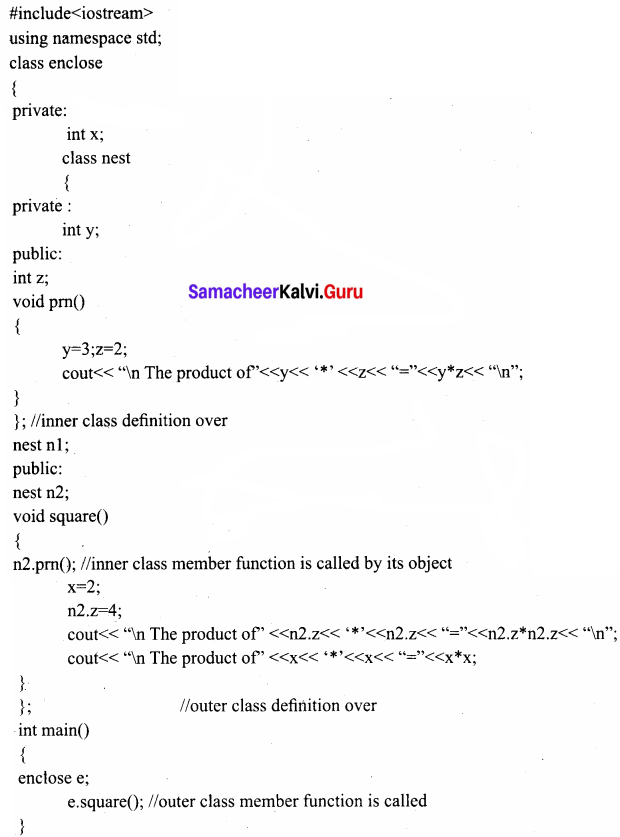Output:
The product of 3*2 = 6
The product of 4*4 = 16
The product of 2*2=4

By declaring an object of a class as a member to another class Whenever an object of a class is declared as a member of another class, it is known as a container class. In the containership the object of one class is declared in another class.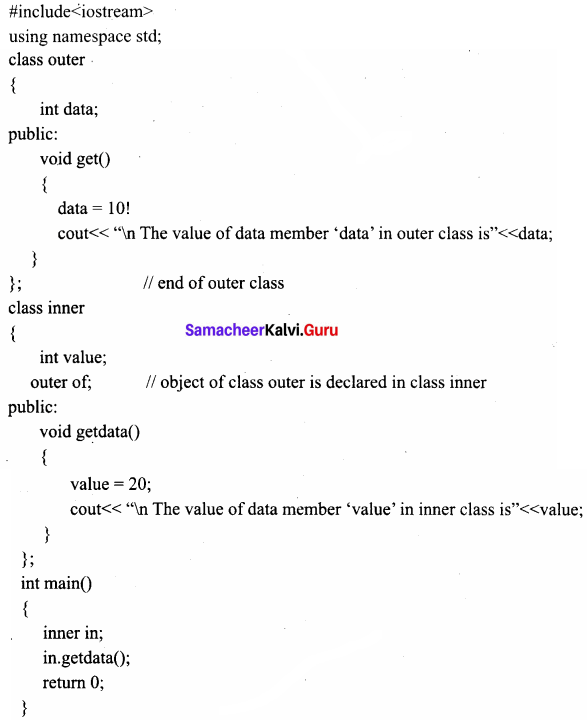Question 2.
Mention the differences between constructor and destructor.
Constructor:

• The name of the constructor must be same as that of the class.
• No return type can be specified for constructor.
• A constructor can have parameter list.
• The constructor function can be overloaded.
• They cannot be inherited but a derived class can call the base class constructor.
• The compiler generates a constructor, in the absence of a user defined constructor.

Destructor:

• The destructor has the same name as that of the class prefixed by the tilde character
• It has no return type.
• The destructor cannot have arguments
• Destructors cannot be overloaded i.e., there can be only one destructor in a class.
• They cannot be inherited.
• In the absence of user defined destructor, it is generated by the compiler.

Question 3.
Define a class RESORT with the following description in C++ :
Private members:

1. Rno // Data member to store room number.
2. Name // Data member to store user name.
3. Charges // Data member to store per day charge.
4. Days //Data member to store the number of days.
5. Compute () // A function to calculate total amount as Days * Charges and if the //total amount exceeds 11000 then total amount is 1.02 * Days ’’’Charges.

Public member:
getinfo() // Function to Read the information like name, room no, charges and days dispinfo () // Function to display all entered details and total amount calculated
//using COMPUTE function
//include
//include
//include
Class RESORT
{

Private:
int Rno;
char name ;
float charges; int days; float compute();
Public:
void getinfoO;
void dispinfo();

}
void RESORT: : getinfo()
{

cout << “Enter Registration number:”; cin >> Rno.;
cout << “\n Enter name:”; gets(name);
cout << “\n Enter per day charges:”; cin >> charges;
cout << “\n Enter number of days:”; cin >> days;

}
void RESORT: : dispinfo()

{

cout << “\n1. Registration number:” << Rno << “\n2. Name:”; puts(name);
cout << “\n3. charges per day:” << charges << “\n4. Number of days:” <<days;
cout<< “\n5. Amount:” << compute();

}
long float;
amount = charges*days;
if (amount> 11000)
amount = 1,02*days*charges;
return amount;
}
RESORT obj;
void main()
{

clrscr();
obj.getinfo();
obj.dispinfoQ;
getch();

}

Question 4.
Write the output of the following.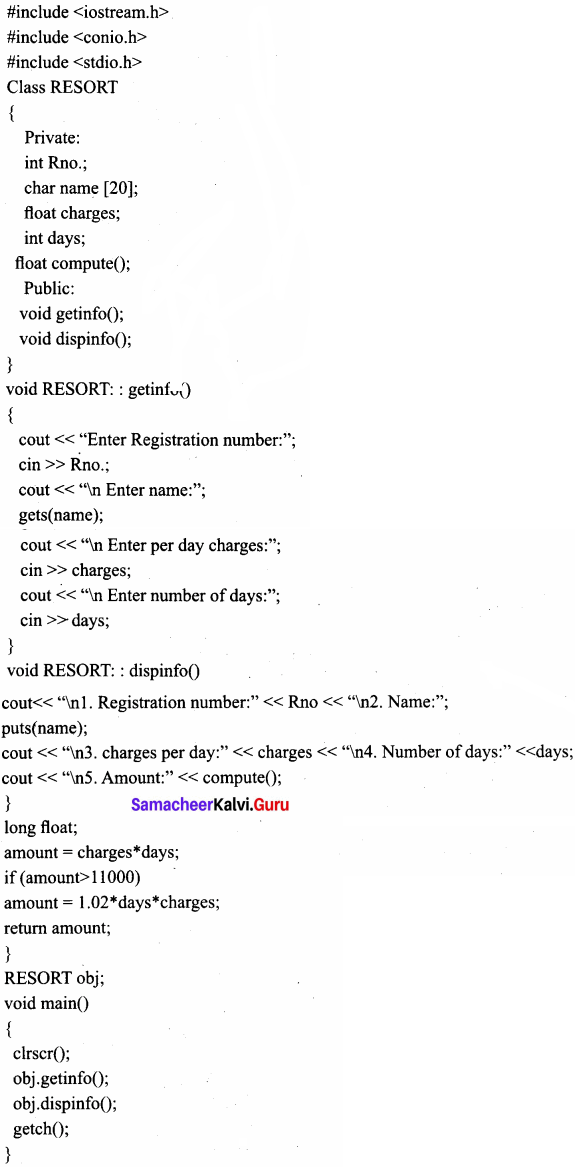Output:
Constructing Subject
Constructing Student
Back to main()
Subject number Days:
Constructing the object
d= 150, Sn= 12
Constructing the object of students
Enter the roll number and the marks secured
mo: 12345 marks: 438
fees: 20000
Back in main()

Question 5.
Write the output of the following.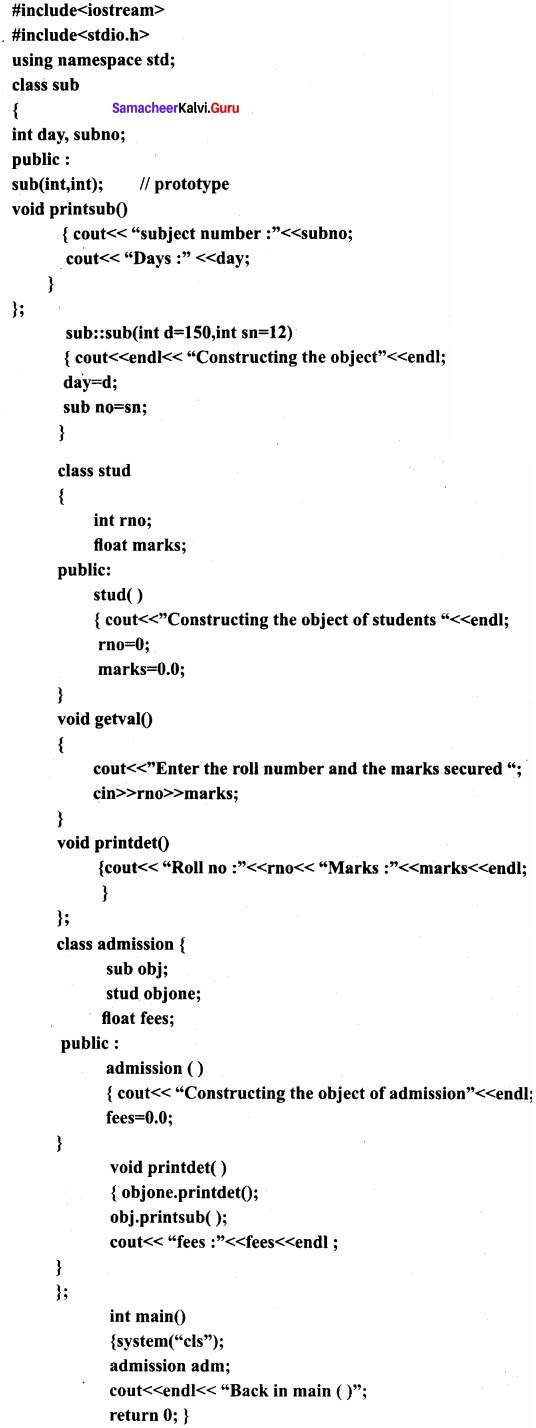Output:
Constructor of class P
Constructor of class P
Constructor of class Q
Constructor of class R
Constructor of class Q
Constructor of class P
Destructor of class P
Destructor of class Q
Destructor of class R
Destructor of class Q
Destructor of class P
Destructor of class P

### Samacheer kalvi 11th Computer Science Arrays and Structures Additional Questions and Answers

PART – 1

Question 1.
The most important feature of C++ is ………………..
(a) object
(b) class
(c) public
(d) All the above
(b) class

Question 2.
Objects are also called as ………………..
(a) instance of class
(b) class
(c) function
(d) scope
(a) instance of class

Question 3.
Calling a member function of an object is also known as ……………….. to object.
(a) call function
(b) call by value
(c) call by reference
(d) sending message
(d) sending messageQuestion 4.
Objects are passed as arguments to a ………………..
(a) call by value
(b) call by reference
(c) member function
(d) global variable
(c) member function

Question 5.
When one class become the member of another class, the relationship is called ………………..
(a) containership
(b) partnership
(c) friendship
(d) all the above
(a) containership

Question 6.
When a class is declared within another class, the inner class is called ……………….. and the outer class is called ………………..
(a) enclosing class, nested class
(b) nested class, enclosing class
(c) first class, second class
(d) A class, B class
(b) nested class, enclosing class

Question 7.
……………….. can be defined either in private or in public section of a class.
(a) Object
(b) Data type
(c) Memory
(d) constructor
(d) constructor

Question 8.
A constructor which can take arguments is called ………………..
(a) parameterised constructor
(b) default constructor
(c) copy constructor
(d) destructor
(a) parameterised constructor

Question 9.
There are ……………….. ways to create an object using parameterized constructor.
(a) 3
(b) 2
(c) 1
(d) 4
(c) 1Question 10.
the name of the symbol is ………………..
(a) hash
(b) arrow
(c) tilde
(d) bracket
(c) tilde

PART – 2

Question 1.
Define methods of a class and write its types.
Class comprises of members. Member functions are called as methods. The member functions of a class can be defined in two ways.

1. Inside the class definition
2. Outside the class definition

Question 2.
What is global object?
If an object is declared outside all the function bodies or by placing their names immediately after the closing brace of the class declaration then it is called as Global object. These objects can be used by any function in the program.

Question 3.
What is called as nesting of member functions?
Only the public members of a class can be accessed by the object of that class, using dot operator. However a member function can call another member function of the same class directly without using the dot operator. This is called as nesting of member functions.

Question 4.
In the absence of user defined constructor, what will the constructor do?
In the absence of user defined constructor the compiler automatically provides the default constructor. It simply allocates memory for the object.Question 5.
What is parameterized constructor?
A constructor which can take arguments is called parameterized constructor.This type of constructor helps to create objects with different initial values. This is achieved by passing parameters to the function.

Question 6.
What will be the output of the following program?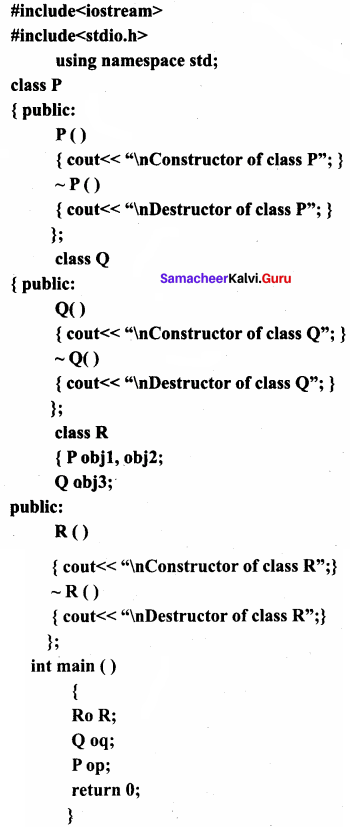Out put:
151515

Question 7.
What will be the output for the following program?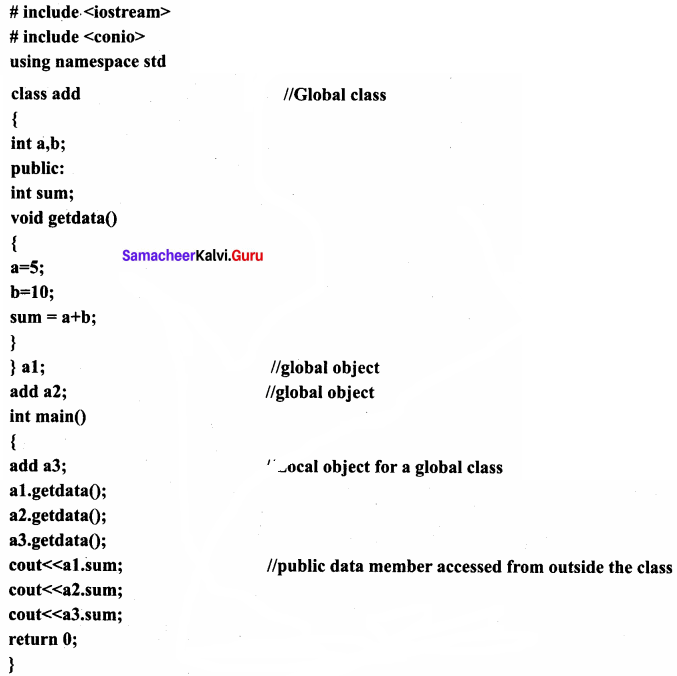PART – 3

Question 1.
Explain local object with an example.
If an object is declared with in a function then it is called local object. It cannot be accessed from outside the function.
# include
# include
using namespace std
{
int a,b; public:
int sum; void
getdata()
{
a = 5; b = 10; sum
= a + b;
}
} a1;
int main()
{
a1.getdata();  //global object
a2.getdata();  //global object
a3.getdata();
cout << a1 .sum;  //Local object for a global class
cout << a2.sum;
cout << a3.sum;
return 0;   //public data member accessed from outside the class
}
Output:
151515

Question 2.
Explain scope resolution operator with an example.
If there are multiple variables with the same name defined in separate blocks then :: (scope resolution) operator will reveal the hidden file scope (global) variable.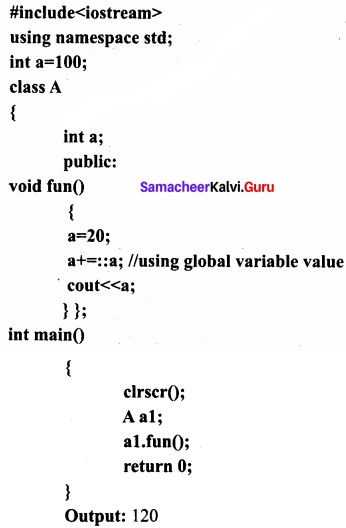Output:
120

Question 3.
What is constructor?
The definition of a class only creates a new user defined data type. The instances of the class type should be instantiated (created and initialized). Instantiating object is done using constructor. An array or a structure in C++ can be initialized during the time of their declaration.

The initialization of class type object at the time of declaration similar to a structure or an array is not possible because the class members have their associated access specifiers (private or protected or public). Therefore Classes include special member functions called as constructors. The constructor function initializes the class object.Question 4.
What are the functions of constructor?
As we know now that the constructor is a special initialization member function of a class that is called automatically whenever an instance of a class is declared or created. The main function of the constructor is

1. To allocate memory space to the object and
2. To initialize the data member of the class object

Question 5.
Explain default constructor with an example.
A constructor that accepts no parameter is called default constructor. For example in the class data program Data ::Data() is the default constructor. Using this constructor objects are created similar to the way the variables of other data types are created.

Example:
int num; //ordinary variable declaration
Data d1; // object declaration

Question 6.
What are the ways to create an object using parameterized constructor?
There are two ways to create an object using parameterized constructor:
Implicit call:
In this method, the parameterized constructor is invoked automatically whenever an object is created. For example simple s1(10, 20); in this for creating the object s1 parameterized constructor is automatically invoked.

Explicit call:
In this method, the name of the constructor is explicitly given to invoke the parameterized constructor so that the object can be created and initialized.

Question 7.
When copy constructor is called?
A copy constructor is called:

1. When an object is passed as a parameter to any of the member functions. Example void simple::putdata(simple x);
2. When a member function returns an object. Example simple getdata() {}
3. When an object is passed by reference to an instance of its own class For example, simplest, s2(s1); // s2(s1) calls copy constructor.

Question 8.
Write the output of the following program.
#include
using namespace std;
class simple
{
private:
int a, b;
public:
simple()
{
a= 0;
b= 0;
cout << “\n Constructor of class – simple”;
}
void getdata()
{
cout << “\n Enter values for a and b (sample data 6 and 7)…”; cin >> a >> b;
}
void putdata()
{
cout << “\nThe two integers are ..” << a << ‘\t’ << b << end1;
cout << “\n The sum of the variables” << a << “+” << b << “=” << a+b; }
~simple()
{ cout << “\n Destructor is executed to destroy the object”;}
};
int main()
{
simple s;
s.getdata();
s.putdata();
return 0;
}
Output:
Constructor of class – simple
Enter values for a and b (sample data 6 and 7)… 6 7
The two integers are .. 6 7
The sum of the variables 6 + 7 = 13
Destructor is executed to destroy the object

PART – 4
IV. Explain in Detail

Question 1.
Create a class called product with the following specifications.
Private members:
code quantity – integer data type
price – float data type
get data() – function to accept values for all data members with no return

Public members:
tax – float
dispdata() member function to display code, quantity, price and tax. The tax is calculated as if the quantity is more than 150, tax is 3000, or else 1000.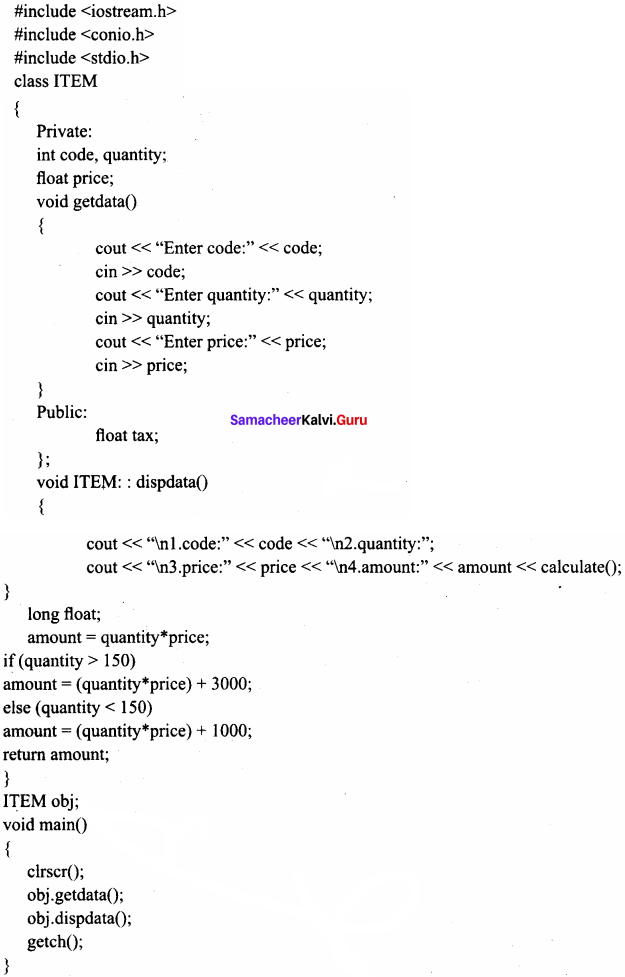Question 2.
Explain pass by value with an example.
When an object is passed by value the function creates its own copy of the object and works on it. Therefore any changes made to the object inside the function do not affect the original object.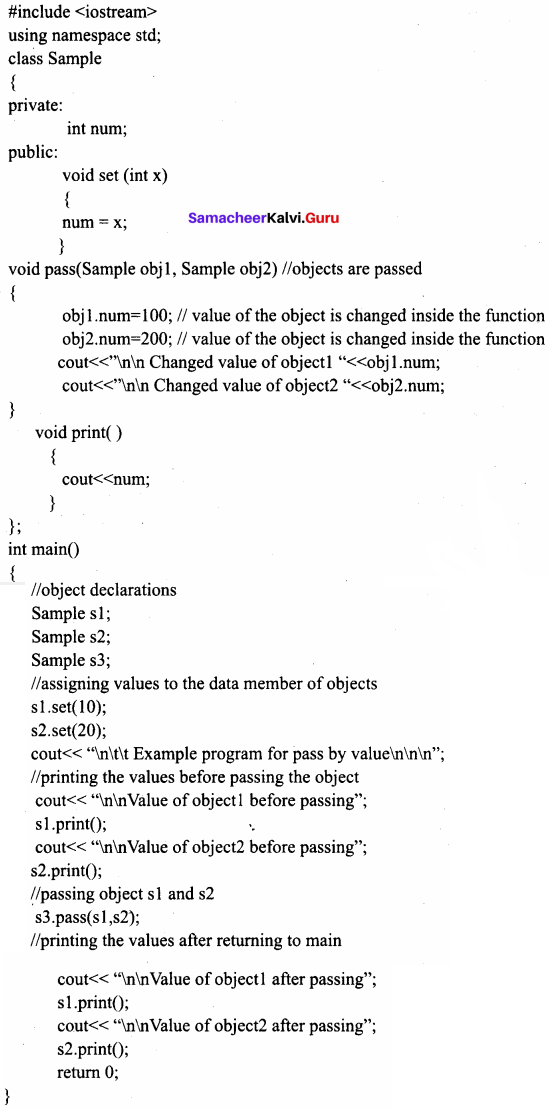Output:
Example program for Pass by value
Value of object 1 before passing 10
Value of object 2 before passing 20
Changed value of object 1 100
Changed value of object 2 200
Value of object 1 after passing 10
Value of object 2 after passing 20

Question 3.
Explain pass by reference with an example.
When an object is passed by reference, its memory address is passed to the function so the called function works directly on the original object used in the function call. So any changes made to the object inside the function definition are reflected in original object.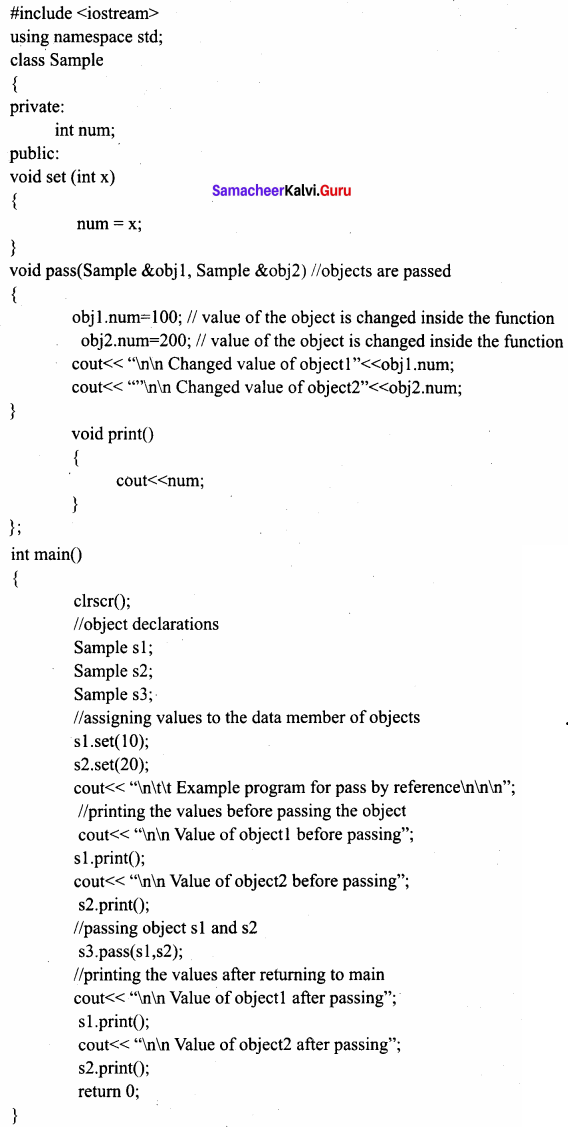Output:
Example program for Pass by reference
Value of object 1 before passing 10
Value of object 2 before passing 20
Changed value of object 1 100
Changed value of object 2 200
Value of object 1 after passing 100
Value of object 2 after passing 200

Question 4.
What are the ways to create an object using parameterized constructor with an example?
There are two ways to create an object using parameterized constructor:
1. Implicit call : In this method, the parameterized constructor is invoked automatically whenever an object is created. For example simple s1( 10,20); in this for creating the object s1 parameterized constructor is automatically invoked.

2. Explicit call : In this method, the name of the constructor is explicitly given to invoke the parameterized constructor so that the object can be created and initialized.

#include
using namespace std;
class simple
{
private:
int a, b;
public:
simple(int m,int n)
{
a = m;
b = n;
cout << “\n Constructor of class – simple invoked for implicit and explicit call” << endl;
}
void putdata()
{
cout << “\n The two integers are…” << a << ‘\t’ << b << endl;
cout << “\n The sum of the variables” << a << “+” << b << “=” << a + b;
}
};
int main()
{
simple s1(10,20); //implicit call
simple s2 = simple(30,45); //explicit call
cout << “\n\t\tObject 1\n”;
s1.putdata();
s2.putdata();
return 0;
}
Output:
Constructor of class – simple invoked for implicit and explicit call
Constructor of class-simple invoked for implicit and explicit call

Object 1
The two integers are… 10 20
The sum of the variables 10 + 20 = 30

Object 2
The two integers are… 30 45.
The sum of the variables 30 + 45 = 75Question 5.
Write the output of the following program.
#include
using namespace std;
class simple
{
private:
int a, b;
public:
simple() //default constructor
{
a = 0; b = 0;
cout << “\n default constructor” << endl;
}
int getdata();
};
int simple :: getdata()
{int tot;
cout << “\n Enter two values”; cin >> a >> b;
}
}
int main()
{
int sum=0; simple s1;
cout << “\n\t\tObject 1 with both values \n”;
for (int i=0;i<3;i++)
sum+=s 1 [i]. getdata();
cout << “\nsum of all object values is” << sum;
return 0;
}
Output:
default constructor
default constructor
default constructor
Object 1 with both values
Enter two values 10 20
Enter two values 30 40
Enter two values 50 50
sum of all object values is 200
}